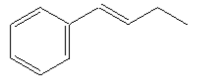# Problem: How many signals would you expect in the 1H NMR spectrum of the following compound? a. 4  b. 5  c. 6  d. 7  e. 8

###### FREE Expert Solution
95% (289 ratings)
###### Problem Details

How many signals would you expect in the 1H NMR spectrum of the following compound?

a. 4
b. 5
c. 6
d. 7
e. 8What scientific concept do you need to know in order to solve this problem?

Our tutors have indicated that to solve this problem you will need to apply the 1H NMR: Number of Signals concept. You can view video lessons to learn 1H NMR: Number of Signals. Or if you need more 1H NMR: Number of Signals practice, you can also practice 1H NMR: Number of Signals practice problems.

What is the difficulty of this problem?

Our tutors rated the difficulty ofHow many signals would you expect in the 1H NMR spectrum of ...as low difficulty.

How long does this problem take to solve?

Our expert Organic tutor, Chris took 3 minutes and 13 seconds to solve this problem. You can follow their steps in the video explanation above.

What professor is this problem relevant for?

Based on our data, we think this problem is relevant for Professor Mohr's class at UIC.